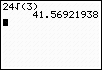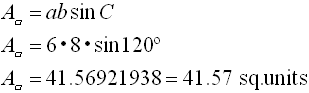Trigonometry Formulas for Area of Triangle
and Area of Parallelogram

 The formula for the area of a triangle is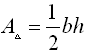where b stands for the base and h stands for the altitude (height) drawn to that base.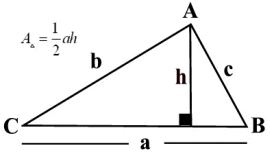(lettering the diagram is of no importance to the formula)

By using trigonometry in the right triangle (on the left side of the diagram), we find:Multiplying by b, gives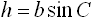.

 Substituting this new value of h into the area formula gives the trig area of triangle formula: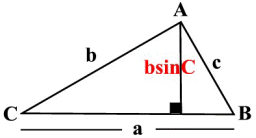SAS Formula for the area of a triangle
where the pattern is to use "
two sides and
the sine of the included angle".

 The diagonal of a parallelogram divides the parallelogram into two congruent triangles.  Consequently, the area of a parallelogram can be thought of as doubling the area of one of the triangles formed by a diagonal.  This gives the trig area formula for a parallelogram: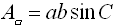Example 1:

Given the triangle at the right, find its area, to the nearest hundredth.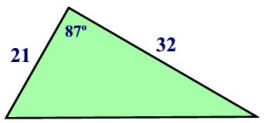Be careful!!!  When using your graphing calculator, be sure that you are in DEGREE Mode, or that you are using the degree symbol if in RADIAN Mode.
 Degree Mode: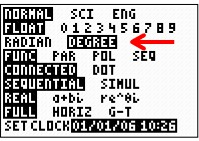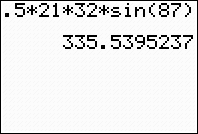Radian Mode: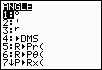Find degree symbol under ANGLE (2nd APPS)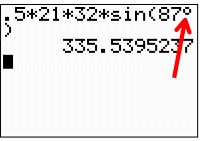Example 2:

Given the parallelogram at the right, find its area to the nearest hundredth.

 Again!!!  Be sure that you are in DEGREE Mode, or that you are using the degree symbol if in RADIAN Mode.

If this problem had asked for an EXACT answer, do not use your calculator, as the calculator rounds the value for sin 120º.  It will be necessary to use the 30º- 60º- 90º reference triangle in Quadrant II.  The EXACT ANSWER will be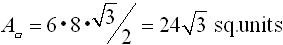Check to see that the exact answer yields the calculator decimal answer.